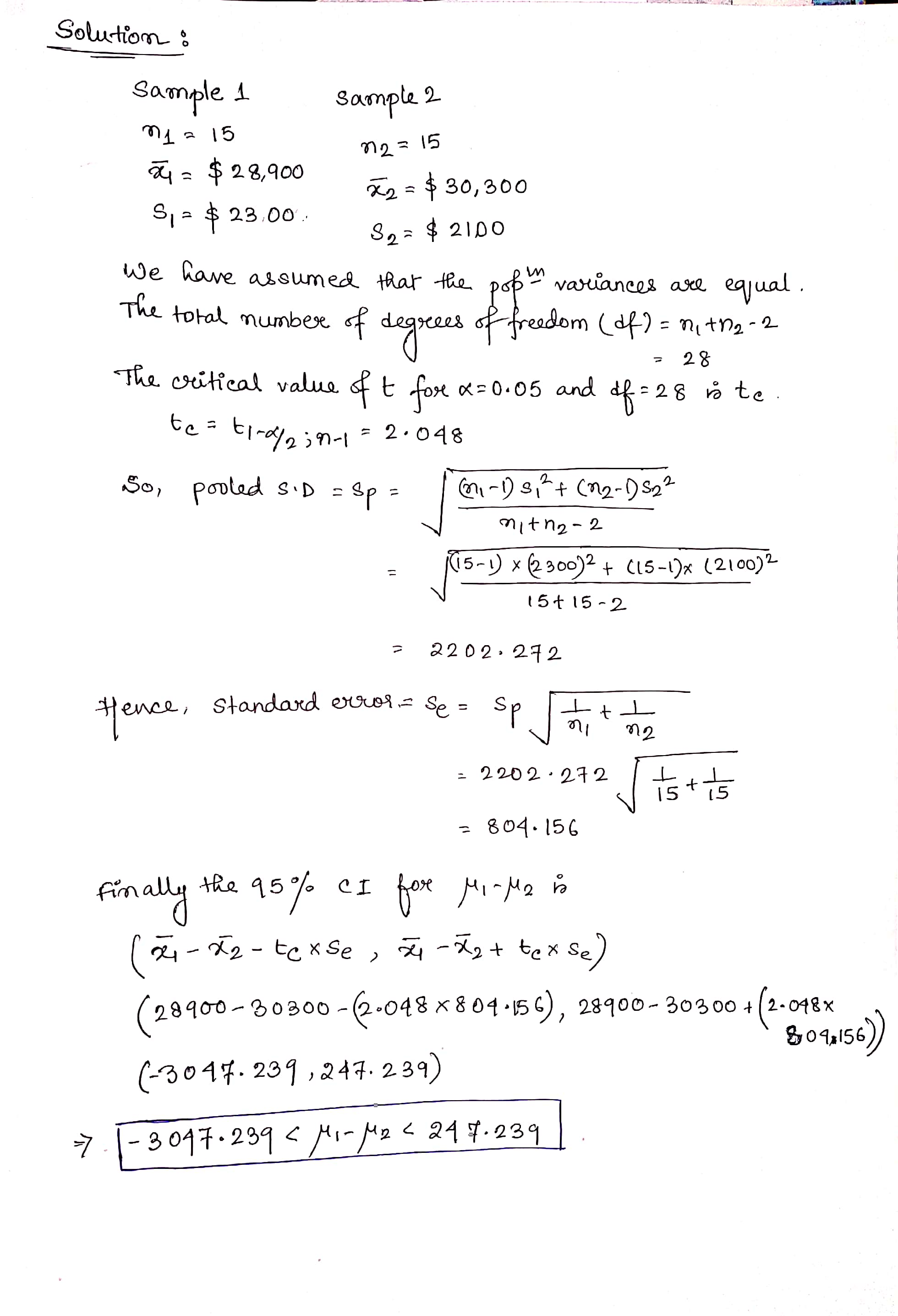##### Question

In: Statistics and Probability

# A study was conducted to determine if the salaries of elementary school teachers from two neighboring...

A study was conducted to determine if the salaries of elementary school teachers from two neighboring districts were equal. A sample of 15 teachers from each district was randomly selected. The mean from the first district was $28,900 with a standard deviation of$2300. The mean from the second district was $30,300 with a standard deviation of$2100. Assume the samples are random, independent, and come from populations that are normally distributed. Construct a 95% confidence interval for μ1 - μ2. Assume that two populations' variance are the same (σ21= σ22).

## Solutions

##### Expert SolutionFor further queries, please comment below.

Thank you.

## Related Solutions

##### A study was conducted to estimate the difference in the mean salaries of elementary school teachers...
A study was conducted to estimate the difference in the mean salaries of elementary school teachers from two neighboring states. A sample of 10 teachers from the Indiana had a mean salary of $28,900 with a standard deviation of$2300. A sample of 14 teachers from Michigan had a mean salary of $30,300 with a standard deviation of$2100. Determine a 95% confidence interval for the difference between the mean salary in Indiana and Michigan.(Assume population variances are different.) *Include...
##### A study was conducted to determine if the salaries of librarians from two neighboring states were...
A study was conducted to determine if the salaries of librarians from two neighboring states were equal. A sample of 100 librarians from each state was randomly selected. The mean from the first state was $29,100 with a standard deviation of$2,200. The mean from the second state was $31,900 with a standard deviation of$2,150. Test the hypothesis that there is no difference in the salaries from both states at the 0.1 significance level. determine the test statistic. Round...
##### A study was conducted to determine if the salaries of librarians from two neighboring cities were...
A study was conducted to determine if the salaries of librarians from two neighboring cities were equal. A sample of 15 librarians from each city was randomly selected. The mean from the first city was​ $28,900 with a standard deviation of​$2300. The mean from the second city was​ $30,300 with a standard deviation of​$2100. Construct a​ 95% confidence interval for mu 1 - mu 2.
##### 2)A study was conducted to determine if the salaries of librarians from two neighboring cities were...
2)A study was conducted to determine if the salaries of librarians from two neighboring cities were equal. A sample of 15 librarians from each city was randomly selected. The mean from the first city was $28,900 with a standard deviation of$2300. The mean from the second city was $30,300 with a standard deviation of$2100.Construct a 95% confidence interval for μ1-μ2. A) Hypothises : B) Critical value (t-critical) : C) Test statistic t-stat and the decision about the test...
##### 2)A study was conducted to determine if the salaries of librarians from two neighboring cities were...
2)A study was conducted to determine if the salaries of librarians from two neighboring cities were equal. A sample of 15 librarians from each city was randomly selected. The mean from the first city was $28,900 with a standard deviation of$2300. The mean from the second city was $30,300 with a standard deviation of$2100.Construct a 95% confidence interval for μ1-μ2. A) Hypothises : B) Critical value (t-critical) : C) Test statistic t-stat and the decision about the test...
##### Salaries for teachers in a particular elementary school district are normally distributed with a mean of...
Salaries for teachers in a particular elementary school district are normally distributed with a mean of $44,000 and a standard deviation of$6,500. We randomly survey ten teachers from that district. 1.Find the probability that the teachers earn a total of over $400,000 2.If we surveyed 70 teachers instead of ten, graphically, how would that change the distribution in part d? 3.If each of the 70 teachers received a$3,000 raise, graphically, how would that change the distribution in part...
##### A researcher claims that the mean of the salaries of elementary school teachers is greater than...
A researcher claims that the mean of the salaries of elementary school teachers is greater than the mean of the salaries of secondary school teachers in a large school district. The mean of the salaries of a random sample of 26 elementary school teachers is $48,250 and the sample standard deviation is$3900. The mean of the salaries of 24 randomly selected secondary school teachers is $45,630 with a sample standard deviation of$5530. At ? = 0.05, can it...
##### Salaries for teachers in a particular elementary school district are normally distributed with a mean of...
Salaries for teachers in a particular elementary school district are normally distributed with a mean of $44,000 and a standard deviation of$6,500. We randomly survey ten teachers from that district. Find the 85th percentile for the sum of the sampled teacher's salaries to 2 decimal places.